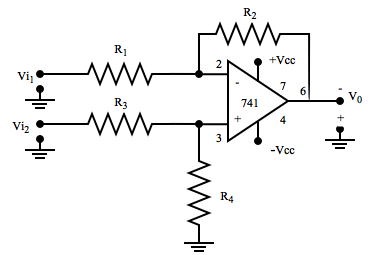MORE IN Elec Circuits & Comm. Fundamentals
MU Computer Engineering (Semester 3)
Elec Circuits & Comm. Fundamentals
December 2016
Total marks: --
Total time: --
INSTRUCTIONS
(1) Assume appropriate data and state your reasons
(2) Marks are given to the right of every question
(3) Draw neat diagrams wherever necessary

1(A) A Mention important specification of ADC and DAC required for communication.
5 M
1(B) A difference amplifier is to be designed to amplify the difference between two voltages by a factor of 20. The inputs each approximately equal to 2V. Determine suitable resistor values for the circuit shown in fig.1 using a 741 opamp.5 M
1(C) With neat blok diagram explain how PLL can be used to generate large number of frequencies from a single reference frequency.
5 M
1(D) When a broadcast AM transmitter is 50% modulated, its antenna current is 12A. What will be current, when the modulation depth is increased to 0.9?
5 M

2(A) For the common source circuit shown in fig.2. Calculate the gate input impedance, the drain output impedance, the circuit input and output impedance and the voltage gain. Use the typical parameters for the FET.
!mage
10 M
2(B) With suitable waveforms explain how op-amp can be used differntiator.
10 M

3(A) Explain the concept of virtual ground in operational amplifier.
5 M
3(B) Compare Hartley and Colpits Oscillator along with neat diagrams.
5 M
3(C) Compare various pulse modulation techniques.
5 M
3(D) Explain the mathematical model for JFET in various regions of operation.
5 M

4(A) Explain the generation of DSBSC using balanced modulator.
10 M
4(B) With neat diagram explain the operating principle of PLL and it's use as a phase shifter.
10 M

5(A) With neat diagram and waveforms, explain the principle of operation of super heterodyne receiver.
10 M
5(B) One input to a conventional AM modulator is a 500 KHz carrier with an amplitude of 20Vp. The second input is 10KHz modulating signal that is of sufficient amplitude to cause a change in the output wave of ± 7.5Vp. Determine
i) upper and lower side frequencies
ii) Modulation coefficient and percentage modulation
iii) peak amplitude of the modulated carrier and upper and lower side frequency voltages
iv) expression for the modulated wave
v) draw the output spectrum
10 M

6(A) Explain the detection of pulse code modulation
5 M
6(B) Discuss delta modulation and adaptive delta modulation.
5 M
6(C) Write short note on generation of FM by Armstrong method
5 M
6(D) Compare n-channel and p-channel JFET with respect to their device features and voltage- current characteristics.
5 M

More question papers from Elec Circuits & Comm. Fundamentals